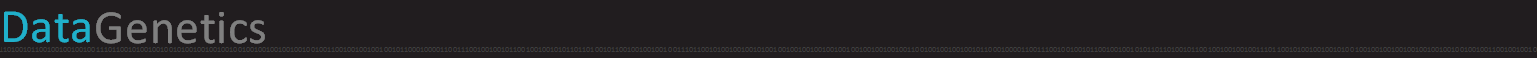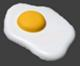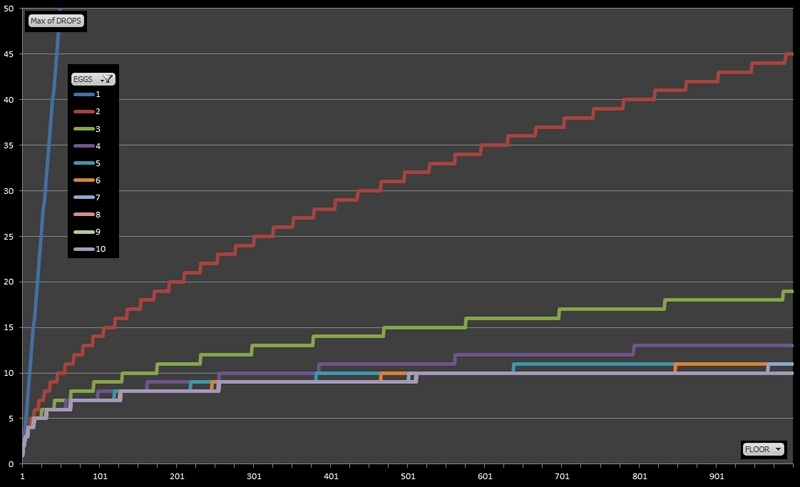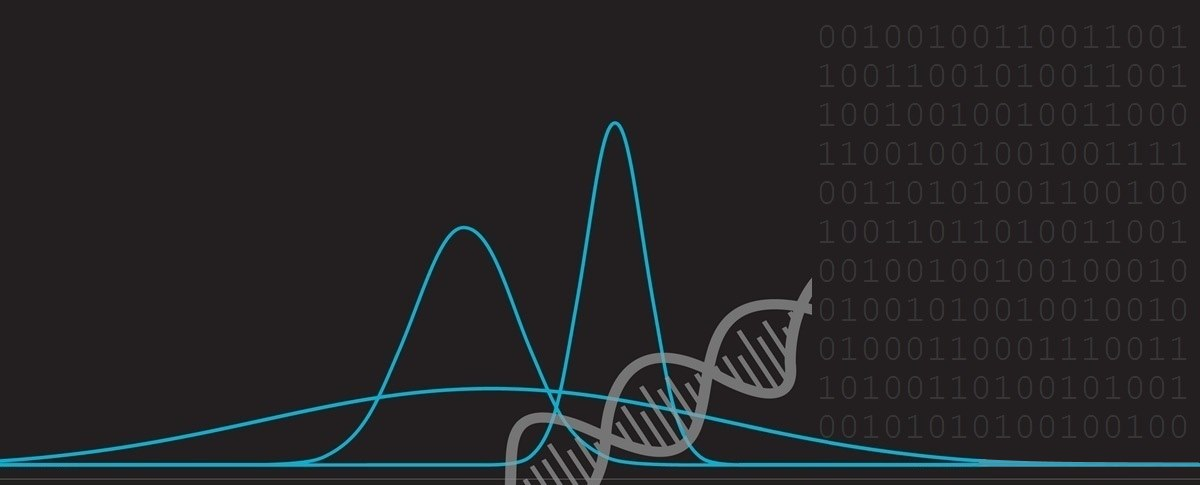The Two Egg ProblemThere’s an interesting mind-teaser/puzzle that floats around the internet in waves. Sometimes it’s described as a Google interview question; sometimes it’s described as a Microsoft interview question. No matter of the origin, it’s a fun little critical thinking puzzle and in this blog posting I’m going to look into it and take it a little further …

Puzzle DefinitionYou are given two eggs, and access to a 100-storey building. Both eggs are identical. The aim is to find out the highest floor from which an egg will not break when dropped out of a window from that floor. If an egg is dropped and does not break, it is undamaged and can be dropped again. However, once an egg is broken, that’s it for that egg. If an egg breaks when dropped from floor n, then it would also have broken from any floor above that. If an egg survives a fall, then it will survive any fall shorter than that. The question is: What strategy should you adopt to minimize the number egg drops it takes to find the solution?. (And what is the worst case for the number of drops it will take?)

There are no tricks, gotchas or other devious ruses. Don’t rat-hole with issues related to terminal velocity, potential energy or wind resistance. This is a math puzzle plain and simple.

Think about the puzzle for a few minutes, and then read on.

One Egg

 Whilst it’s not strictly part of the puzzle, let’s first imagine what we’d do if we had only one egg. Once this egg is broken, that’s it, no more egg. So, we really have no other choice but to start at floor 1. If it survives, great, we go up to floor 2 and try again, then floor 3 … all the way up the building; one floor at a time. Eventually the egg will break* and we’ll have a solution. For example, if it breaks on floor 57, we know that the highest floor that an egg can withstand a drop from is floor 56. There’s no other one egg solution. Sure, if we’d been feeling lucky we could have gone up the floors in two’s but imagine if the egg broke on floor 16; we have no way of knowing if it would have also broken on floor 15!* Of course there is a chance that the egg will not break on floor 100, and this is a valid solution.Many EggsAt the other extreme, what if we had an infinite number of eggs? (Or at least as many eggs as we need). What would our strategy be here? In this case we’d use one of a programmer’s favorite tools, the binary tree. First we’d go to floor 50 and drop an egg. It either breaks, or it does not. The outcome of this drop instantly cuts our problem in half. If it breaks, we know the solution lives in the bottom half of the building (floor 1 – floor 49). If it survives, we know the solution is in the top half of the building (floor 51 – 100). On each drop, we keep dividing the problem in half and half again until we get to our solution. The mathematicians in the audience will quickly see that the number of drops required for this solution is log2 n, where n is the number of floors of the building. (This is like asking how many powers of two there are). Because this building does not have a number of floors equal to a round number power of two, we need to round up to number of drops to get seven ( log2 100 = 6.644 ) (Using seven drops, we could solve this problem any building up to 128 floors. Eight drops would allow us to solve the puzzle for a building with twice the height at 256 floors …) Depending on the final answer, the actual number of eggs broken using a binary search will vary. At worst it will be seven eggs (if the floor is actually floor 1, since every drop will break the egg). At best it will be no eggs (if the actually answer is floor 100, since the egg will survive every drop made). (There's a very interesting lesson here which I'll cover in a future posting: Understanding the difference between Expected Answer and Worst Case Answer. These can be very different things!)

Back to two eggs

OK, let’s get back to the original two egg problem. As we’ve seen from above, the worst case using a binary search would break seven eggs; not acceptable when we only have two eggs.

It does not take much imagination to see why a binary search solution will not work (optimally) for two eggs. Let’s imagine we did try a binary search and dropped our first egg from floor 50. If it broke, we’d be instantly reduced to a one egg problem, so then we would have to start with our last egg from floor 1 and keep going up one floor at a time until that breaks. As a worst case, it will take us 50 drops. We can do better …

 What happens if we started off with our first egg going up by floors ten at a time? We can start dropping our egg from floor 10; if it survives we try floor 20, then floor 30 … we carry on until the first egg breaks. Once we’ve broken our first egg we know that the solution must lay somewhere in the nine floors just below, so we back off nine floors and step through these floors one at a time until we find a solution.This is certainly an improvement, but what is our worst case with this strategy? Well, imagine we dropped eggs on every 10th floor all the way up, and our first egg broke on floor 100. This has taken us ten drops so far. Now we know the solution must lay somewhere between floor 91 and floor 99 and we have to go through these in ones, starting at floor 91. The worst case would be if the solution was on floor 99, and this would take us nine more drops to determine. The worst case therefore, if we go by tens, is 19 drops.This is a huge improvement, but can we do better?Thinking about the 10 floor strategy again we can see that, whilst our worst case is 19 drops, some other possible solutions will take less than this (for instance, if the first egg broke on floor 10 then, at worst, from here we only have to make nine more drops to find the solution). Knowing that, what if we dropped our first egg from floor 11 instead? If the egg breaks on this floor, it will still only take ten more drops to find the solution (and if it doesn’t break, great, we’ve eliminated more floors than before! win-win?) Let's follow this idea, and see where it leads …Well, how about if we dropped our first egg from floor 12 then? A similar argument to above; if it breaks, we only have to try eleven floors with the second egg. If it doesn’t break, we can step up another dozen floors, and so on … But hold on a minute! … If first we try floor 12, then 24, then 36, then 48, 60, 72, 84, 96 then it finally breaks, we’ve wasted eight drops already, and we still have to check (up to) eleven more floors with our second egg, so we’re back at a worst case of 19 drops. Problems where the solution lays lower down the building are taking less drops than when the solution lays higher up. We need to come up with a strategy that makes things more uniform.

Minimization of Maximum Regret

 What we need is a solution that minimizes our maximum regret. The examples above hint towards what we need is a strategy that tries to make solutions to all possible answers the same depth (same number of drops). The way to reduce the worst case is to attempt to make all cases take the same number of drops.As I hope you can see by now, if the solution lays somewhere in a floor low down, then we have extra-headroom when we need to step by singles, but, as we get higher up the building, we’ve already used drop chances to get there, so there we have less drops left when we have to switch to going floor-by-floor.

Let’s break out some algebra.

Imagine we drop our first egg from floor n, if it breaks, we can step through the previous (n-1) floors one-by-one.

If it doesn’t break, rather than jumping up another n floors, instead we should step up just (n-1) floors (because we have one less drop available if we have to switch to one-by-one floors), so the next floor we should try is floor n + (n-1)

Similarly, if this drop does not break, we next need to jump up to floor n + (n-1) + (n-2), then floor n + (n-1) + (n-2) + (n-3)

We keep reducing the step by one each time we jump up, until that step-up is just one floor, and get the following equation for a 100 floor building:

n + (n-1) + (n-2) + (n-3) + (n-4) + … + 1  >=  100

This summation, as many will recognize, is the formula for triangular numbers (which kind of makes sense, since we’re reducing the step by one each drop we make) and can be simplified to:

n (n+1) / 2  >=  100

This is a quadratic equation, with the positive root of 13.651 (Which we have to round up to 14. This is not a John Malkovich movie!).

Two egg solution

We now have all the information to compute the optimal two egg solution.

Drop  Floor
#114
#227
#339
#450
#560
#669
#777
#884
#990
#1095
#1199
#12100

Our first drop should be from floor 14, if egg survives we step up 13 floors to floor 27, then up 12 floors to 39 …

The optimal strategy is to work our way through the table until the first egg breaks, then back up to one floor higher than the line above and then proceed floor-by-floor until we find the exact solution.

This complete table is shown in here on the left.

The maximum of 14 drops is a combination first egg drops (made in steps), and second egg drops (made in ones). For every drop we take hopping up the tower, we reduce the worst-case number of single drops we’d have to take so that no solution is an outlier.

“Look see I can do three” ** “Ten Apples Up On Top” – Dr. Seuss

Why stop at two eggs? What is the optimal strategy if we had three eggs? (Dare I say four? five? …)

Things get more complex with three eggs, but taking a deep breath we can apply the same principle of minimizing maximum regret. We need to select our first egg such that, if it breaks, or does not break, it results in a problem, which recursively is now a two egg problem, that we already know how to solve to minimize maximum regret!

OK hold on tight, here we go …

Let's define our building to have a maximum of k floors. Let's say that the number of egg we have is represented by e, and the floor we are currently attempting to drop from is represented by n. With me so far? OK, what we need to find our optimal solution is to calculate what would happen if we dropped an egg from every floor (1 through to k) and (recursively) calculate the minimum number of droppings needed in the worst case. We're looking for the floor that gives the minimum solution to the worst case.

If we drop an egg from floor n, one of two events happens:

1) The egg breaks, so now our problem is reduced to a tower of height n-1, and we now have e-1 eggs.

2) The egg doesn't break and now we need to check k-n floors and we still have e eggs.

Remember we need to mimimize the number of drops in the worst case, so we take the higher (max) of these two situations, and select the floor which yields the minimum number of drops.

The code to solve this is fairly easy to write recursively, but suffers from a common problem that occurs with recursive solutions in which the same sub-problems are evaluated again, and again, and again, dragging performance to a grind for anything other than trivial solutions. To get around this, we need to keep track of values already computed so that we don't have to repeat the calculation.

Rather than getting lost in gnarly code, let's just share some of the results, and for some buildings higher than 100 stories.

Lots of of eggs, and lots of floorsHere's a table showing the worst case number of drops it would take for a variety of floors with anywhere from 1 – 10 eggs.

Eggs
Building Height  1  2  3  4  5  6  7  8  9  10
1 floor1111111111
2 floors2222222222
3 floors3222222222
4 floors4333333333
5 floors5333333333
6 floors6333333333
7 floors7433333333
8 floors8444444444
9 floors9444444444
10 floors10444444444
11 floors11544444444
12 floors12544444444
13 floors13544444444
14 floors14544444444
15 floors15554444444
16 floors16655555555
17 floors17655555555
18 floors18655555555
19 floors19655555555
20 floors20655555555
21 floors21655555555
22 floors22755555555
23 floors23755555555
24 floors24755555555
25 floors25755555555
30 floors30865555555
35 floors35866666666
40 floors40966666666
45 floors45976666666
50 floors501076666666
100 floors1001498777777
200 floors20020119888888
300 floors300241310999999
400 floors4002814111099999
500 floors50032151110109999
1,000 floors1000451913111111101010

A graphs is worth a thousand floors

Here's the data plotted in graphical form. The y-axis represents the worst case number of drops needed to find the solution. The x-axis represents the number of floors in the building being tested (from 1 – 1000). There is a distinct line for each number of eggs.

[CLICK GRAPH FOR LARGER VERSION]The blue line, representing the one egg solution, has a gradient of 1 and for every building, the worst case number of drops is the same as the number of floors.

Adding an extra egg to make two eggs makes a huge difference. Adding a third egg makes a smaller difference again.

For small numbers of floors and larger numbers of eggs, the solutions merge as we end up having excess eggs and the solution simplifies to that of the binary search as we discussed at the beginning of this article.That's an awful lot of omelettes!

Check out other interesting blogarticles. (No eggs were hurt in the creation of this article).

You can find a complete list of all the articles here.Click here to receive email alerts on new articles.